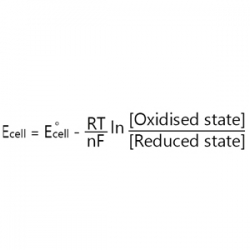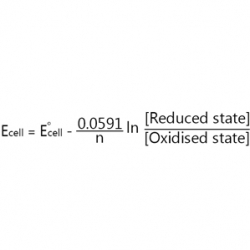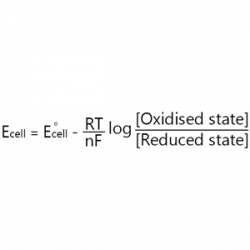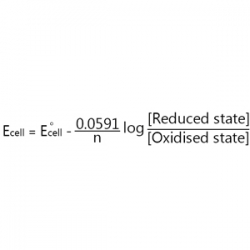Determination of EMF of a Cell
 1) What is an electrochemical cell? Device for converting mechanical energy into chemical energy. Device for converting chemical energy into mechanical energy. Device for converting chemical energy into electrical energy. Device for converting electrical energy into chemical energy.

 2) Which one of the following is the correct representation of a cell? A⁺|A||B|B⁺ A⁺|A||B⁺|B A|A⁺||B⁺|B A|A⁺||B|B⁺

 3) Which one of the following is not used as an electrolyte in salt bridge? KCl KNO₃ NH₄NO₃ CH₃COOH4)Which one of the following is the correct representation of Nernst equation?5) Electrode potential of an electrochemical cell depends on which of the following parameters? Temperature Standard electrode potential Concentration of the reacting species All of these

 6) The standard reduction potential of three metallic cations A, B & C are 0.52, -3.30 & -1.18 V respectively. The order of reducing powers of the corresponding metal is C > B > A C > A > B A > B > C B > C > A

 7) What is the significance of a salt bridge? It prevents liquid junction potential. It completes the electrical circuit by connecting the electrolytes in the two half cells. It maintains the electrical neutrality of the solutions in the two half cells. All of these.

 8) E° X⁺|X, E° Y⁺|Y. E° Z³⁺|Z, E° B²⁺|B are -3.05, -2.71, -1.66, -0.13. Which is the strongest oxidizing agent? Z³⁺ X⁺ Y⁺ B²⁺

 9) The standard reduction potentials of Cu²⁺|Cu and Cu²⁺|Cu⁺ are 0.337 V and 0.153 V respectively. What is the standard electrode potential of Cu⁺|Cu? 0.49 0.454 0.184 0.051

 10) What is the potential of a half cell consisting of zinc electrode in 0.01 M ZnSO₄ solution at 25°C? (E° = 0.763 V) 0.8223 0.703 0.8221 0.604

Cite this Simulator: